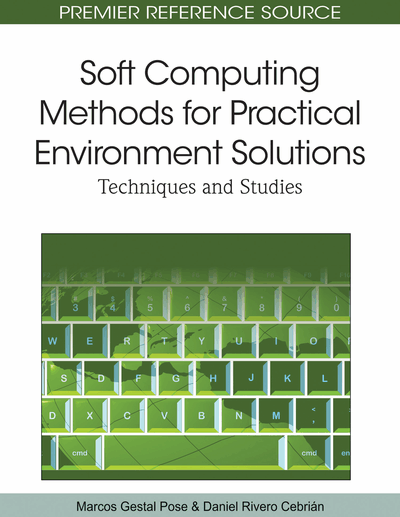# Characterization and Modelization of Surface Net Radiation through Neural Networks

Antonio Geraldo Ferreira (University of Valencia, Spain & Fundação Cearense de Meteorologia e Recursos Hídricos (FUNCEME), Brazil), Emilio Soria (University of Valencia, Spain), Antonio J. Serrano López (University of Valencia, Spain) and Ernesto Lopez-Baeza (University of Valencia, Spain)
Copyright: © 2010 |Pages: 18
DOI: 10.4018/978-1-61520-893-7.ch016
OnDemand PDF Download:
Available
\$37.50
No Current Special Offers

## Abstract

Artificial neural networks have shown to be a powerful tool for system modeling in a wide range of applications. In this chapter, the focus is on neural network applications to obtain qualitative/quantitative relationships between meteorological and soil parameters and net radiation, the latter being a significant term of the surface energy balance equation. By using a Multilayer Perceptron model an artificial neural network based on the above mentioned parameters, net radiation was estimated over a vineyard crop. A comparison has been made between the estimates provided by the Multilayer Perceptron and a linear regression model that only uses solar incoming shortwave radiation as input parameter. Self-Organizing Maps, another type of neural model, made it possible to get knowledge in an easy way on how the input variables are related to each other in the data set. The results achieved show the potential of artificial neural networks as a tool for net radiation estimation using more commonly measured meteorological parameters.
Chapter Preview
Top

## Background

The energy exchanges between the land surface and the atmosphere can be described by the surface energy balance equation given by the algebraic sum of fluxes over the surfaceQ* + QH + QλE + QG = 0 (1) where Q* is net radiation at surface, QH is the sensible heat flux, QλE is the latent heat flux, a product of the evaporative rate E and the latent heat per unit quantity of water evaporated, λ, and QG is the soil heat flux, the rate at which heat is transferred from the surface downward into the soil profile, all in units of W m-2. In Eq. (1), the fluxes are considered as positive if directed toward the surface and negative in the opposite case (Hillel, 2004) (See Figure 1).

Figure 1.

Typical variation of terms of the surface energy balance for: (a) daytime over land and (b) night-time over land. The arrow size indicates the relative magnitude

In Eq. (1), Q* is also the algebraic sum of net components of shortwave (K*) and longwave (L*) radiation, which can be written asQ* = K* + L*(2) where the symbol * represents net flux. The K* and L* in Eq. (2) can be written asK* = K↓ - K↑(3) andL* = L↓ - L↑(4) finally resultingQ* = K↓ - K↑ + L↓ - L↑(5) where the downward arrows (↓) and upward arrows (↑) indicate incoming and outgoing radiation components respectively, and the energy moving toward the surface is also considered positive and the energy moving away from the surface is considered negative.

## Complete Chapter List

Search this Book:
Reset